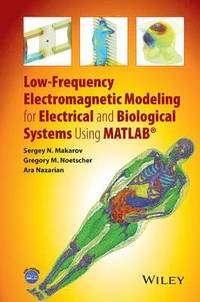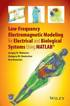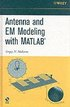Fler böcker inom
Format
Inbunden (Hardback)
Språk
Engelska
Antal sidor
616
Utgivningsdatum
2015-06-12
Upplaga
1
Förlag
Wiley-Blackwell
Dimensioner
241 x 165 x 38 mm
Vikt
975 g
Antal komponenter
1
ISBN
9781119052562

### Du kanske gillar# Low-Frequency Electromagnetic Modeling for Electrical and Biological Systems Using MATLAB

Inbunden Engelska, 2015-06-12
1459
Skickas inom 5-8 vardagar.
Fri frakt inom Sverige för privatpersoner.
Finns även som
1959
1959
Provides a detailed and systematic description of the Method of Moments (Boundary Element Method) for electromagnetic modeling at low frequencies and includes hands-on, application-based MATLAB(R) modules with user-friendly and intuitive GUI and a highly visualized interactive output. Includes a full-body computational human phantom with over 120 triangular surface meshes extracted from the Visible Human Project(R) Female dataset of the National library of Medicine and fully compatible with MATLAB(R) and major commercial FEM/BEM electromagnetic software simulators. This book covers the basic concepts of computational low-frequency electromagnetics in an application-based format and hones the knowledge of these concepts with hands-on MATLAB(R) modules. The book is divided into five parts. Part 1 discusses low-frequency electromagnetics, basic theory of triangular surface mesh generation, and computational human phantoms. Part 2 covers electrostatics of conductors and dielectrics, and direct current flow. Linear magnetostatics is analyzed in Part 3. Part 4 examines theory and applications of eddy currents. Finally, Part 5 evaluates nonlinear electrostatics. Application examples included in this book cover all major subjects of low-frequency electromagnetic theory. In addition, this book includes complete or summarized analytical solutions to a large number of quasi-static electromagnetic problems. Each Chapter concludes with a summary of the corresponding MATLAB(R) modules. * Combines fundamental electromagnetic theory and application-oriented computation algorithms in the form of stand alone MATLAB(R) modules * Makes use of the three-dimensional Method of Moments (MoM) for static and quasistatic electromagnetic problems * Contains a detailed full-body computational human phantom from the Visible Human Project(R) Female, embedded implant models, and a collection of homogeneous human shells Low-Frequency Electromagnetic Modeling for Electrical and Biological Systems Using MATLAB(R) is a resource for electrical and biomedical engineering students and practicing researchers, engineers, and medical doctors working on low-frequency modeling and bioelectromagnetic applications.

## Passar bra ihop

1.2. +
3.De som köpt den här boken har ofta också köpt Antenna and EM Modeling with MATLAB av Sergey N Makarov (inbunden).

## Kundrecensioner

Har du läst boken? Sätt ditt betyg »

## Övrig information

Sergey N. Makarov is a Professor in the Department of Electrical and Computer Engineering at Worcester Polytechnic Institute (WPI). Gregory M. Noetscher is a Senior Research Electrical Engineer at the U.S. Army Natick Soldier Research, Development and Engineering Center (NSRDEC) in Natick, MA. Ara Nazarian is an Assistant Professor of Orthopaedic Surgery, Harvard Medical School, Center for Advanced Orthopaedic Studies, Beth Israel Deaconess Medical Center (BIDMC).

## Innehållsförteckning

PREFACE xi ACKNOWLEDGMENTS xv ABOUT THE COMPANION WEBSITE xvii PART I LOW-FREQUENCY ELECTROMAGNETICS.COMPUTATIONAL MESHES.COMPUTATIONAL PHANTOMS 1 1 Classification of Low-Frequency Electromagnetic Problems. Poisson and Laplace Equations in Integral Form 3 Introduction 3 1.1 Classification of Low-Frequency Electromagnetic Problems 4 1.2 Poisson and Laplace Equations Boundary Conditions and Integral Equations 18 References 30 2 Triangular Surface Mesh Generation and Mesh Operations 35 Introduction 35 2.1 Triangular Mesh and its Quality 36 2.2 Delaunay Triangulation. 3D Volume and Surface Meshes 46 2.3 Mesh Operations and Transformations 56 2.4 Adaptive Mesh Refinement and Mesh Decimation 75 2.5 Summary of MATLAB(R) Scripts 81 References 85 3 Triangular Surface Human Body Meshes for Computational Purposes 89 Introduction 89 3.1 Review of Available Computational Human Body Phantoms and Datasets 92 3.2 Triangular Human Body Shell Meshes Included with the Text 96 3.3 VHP-F Whole-Body Model Included with the Text 108 References 126 PART II ELECTROSTATICS OF CONDUCTORS AND DIELECTRICS. DIRECT CURRENT FLOW 131 4 Electrostatics of Conductors. Fundamentals of the Method of Moments. Adaptive Mesh Refinement 133 Introduction 133 4.1 Electrostatics of Conductors. MoM (Surface Charge Formulation) 134 4.2 Gaussian Quadratures. Potential Integrals. Adaptive Mesh Refinement 147 4.3 Summary of MATLAB(R) Modules 162 References 167 5 Theory and Computation of Capacitance. Conducting Objects in External Electric Field 169 Introduction 169 5.1 Capacitance Definitions: Self-Capacitance 170 5.2 Capacitance of Two Conducting Objects 180 5.3 Systems of Three Conducting Objects 188 5.4 Isolated Conducting Object in an External Electric Field 196 5.5 Summary of MATLAB(R) Modules 204 References 212 6 Electrostatics of Dielectrics and Conductors 215 Introduction 215 6.1 Dielectric Object in an External Electric Field 216 6.2 Combined Metal Dielectric Structures 229 6.3 Application Example: Modeling Charges in Capacitive Touchscreens 239 6.4 Summary of MATLAB(R) Modules 245 References 253 7 Transmission Lines: Two-Dimensional Version of the Method of Moments 257 Introduction 257 7.1 Transmission Lines: Value of the Electrostatic Model Analytical Solutions 258 7.2 The 2D Version of the MoM for Transmission Lines 273 7.3 Summary of MATLAB(R) Modules 284 References 287 8 Steady-State Current Flow 289 Introduction 289 8.1 Boundary Conditions. Integral Equation. Voltage and Current Electrodes 290 8.2 Analytical Solutions for DC Flow in Volumetric Conducting Objects 300 8.3 MoM Algorithm for DC Flow. Construction of Electrode Mesh 311 8.4 Application Example: EIT 320 8.5 Application Example: tDCS 327 8.6 Summary of MATLAB(R) Modules 336 References 341 PART III LINEAR MAGNETOSTATICS 347 9 Linear Magnetostatics: Surface Charge Method 349 Introduction 349 9.1 Integral Equation of Magnetostatics: Surface Charge Method 350 9.2 Analytical versus Numerical Solutions: Modeling Magnetic Shielding 358 9.3 Summary of MATLAB(R) Modules 367 References 369 10 Inductance. Coupled Inductors. Modeling of a Magnetic Yoke 371 Introduction 371 10.1 Inductance 372 10.2 Mutual Inductance and Systems of Coupled Inductors 385 10.3 Modeling of a Magnetic Yoke 404 10.4 Summary of MATLAB(R) Modules 415 References 421 PART IV THEORY AND APPLICATIONS OF EDDY CURRENTS 423 11 Fundamentals of Eddy Currents 425 Introduction 425 11.1 Three Types of Eddy Current Approximations 426 11.2 Exact Solution for Eddy Currents without Surface Charges Created by Horizontal Loops of Current 440 11.3 Exact Solution for a Sphere in an External AC Magnetic Field 453 11.4 A Simple Approximate Solution for Eddy Currents in a Weakly Conducting Medium 460 11.5 Summary of MATLAB(R) Modules 464 References 470 12 Computation of Eddy Currents via the Surface Charge Method 473 Introduction 473 12.1 Numerical Solution in a Weakly Cond